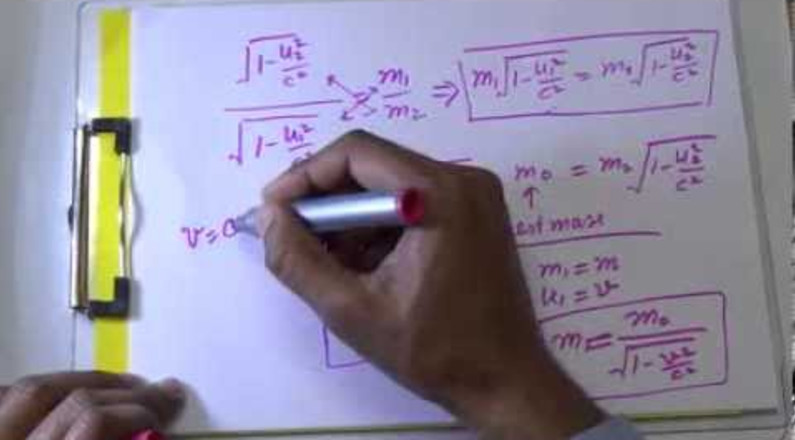In classical mechanics, mass of a particle is considered to be a constant quantity and independent of its velocity. However in relativistic mechanics the length, time and mass also depends on the relative velocity.Suppose two similar balls B1 and B2 we have, velocity (u’ and -u’) and masses of its are similar in S’ frame. These two balls collide with each other in such a way that it convert into a single body. Now, apply the law of conservation of linear momentum.

for more information